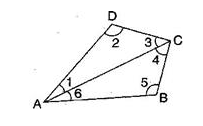# what is the sum of the …## CBSE, JEE, NEET, NDA

Question Bank, Mock Tests, Exam Papers

NCERT Solutions, Sample Papers, Notes, Videos

what is the sum of the measures of the angles of a convex quadrilateral?will this property hold if the quadrilateral is not convex?(make a non-convex quadrilateral and try)

Preeti Dabral 1 month, 1 week ago

Let ABCD is a convex quadrilateral, then we draw a diagonal AC which divides the quadrilateral in two triangles.
{tex}\angle \mathrm{A}+\mathrm{B}+\angle \mathrm{C}+\angle \mathrm{D}{/tex}
{tex}\angle 1+\angle 6+\angle 5+\angle 4+\angle 3+\angle 2{/tex}
{tex}(\angle 1+\angle 2+\angle 3)+(\angle 4+\angle 5+\angle 6){/tex}{tex}180^{\circ}+180^{\circ}{/tex} [By Angle sum property of triangle]
{tex}360^{\circ}{/tex}
Hence, the sum of measures of the triangles of a convex quadrilateral is {tex}360^{\circ}{/tex}.
Yes, if quadrilateral is not convex then, this property will also be applied.

Related Questions:## myCBSEguide

Trusted by 1 Crore+ Students

#### CBSE Test Generator

Create papers in minutes

Print with your name & Logo

3 Lakhs+ Questions

Solutions Included

Based on CBSE Blueprint

Best fit for Schools & Tutors

#### Work from Home

• Work from home with us
• Create questions or review them from home

No software required, no contract to sign. Simply apply as teacher, take eligibility test and start working with us. Required desktop or laptop with internet connection